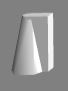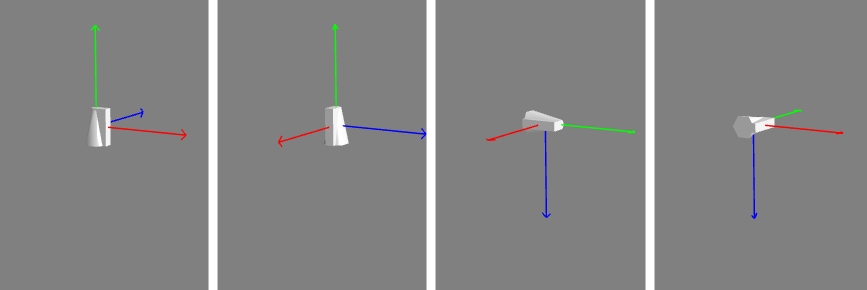# Mesh Rotation

## Rotation

Rotation in 3D space is always tricky. The order in which rotations are applied and the frame of reference used changes the final orientation of a mesh. There is a range of conventions for applying rotations in 3D modelling. More on those later in this section.

To help in visualizing orientation this asymmetric mesh is used in examplesThe most straight forward method to change the orientation of a mesh is the rotation property.

mesh.rotation = new BABYLON.Vector3(alpha, beta, gamma); //alpha, beta, gamma in radians

or

mesh.rotation.x  =  alpha; //rotation around x axismesh.rotation.y  =  beta;  //rotation around y axismesh.rotation.z  =  gamma; //rotation around z axis

where alpha, beta, and gamma are angles measured in radians are known as the Euler angles.

Four questions need an immediate answer

1. Where is the center of rotation?
2. Are they applied in a clockwise or counter clockwise direction?
3. What is the frame of reference?
4. In which order are they applied?

The first two are easy to answer since, on creation, the center of rotation is the local origin of the mesh and rotations are always counter clockwise when looking in the positive direction of the stated axis.

The answer to 4 depends on 3 and in Babylon.js the rotation frame of reference is in the local space of the mesh being rotated. It makes no difference which one of the following four sets of code you use the resulting orientation will always be the same.

mesh.rotation = new BABYLON.Vector3(alpha, beta, gamma);
mesh.rotation.x  =  alpha;mesh.rotation.y  =  beta;mesh.rotation.z  =  gamma;
mesh.rotation.z  =  gamma;mesh.rotation.x  =  alpha;mesh.rotation.y  =  beta;
mesh.rotation.y  =  beta;mesh.rotation.z  =  gamma;mesh.rotation.x  =  alpha;

The order is - a rotation of beta about the local y axis, then alpha about the local x axis and finally a rotation of gamma about the local z axis.

The following sequence of images shows this order of rotation.
Red x axis, green y axis, blue z axis.